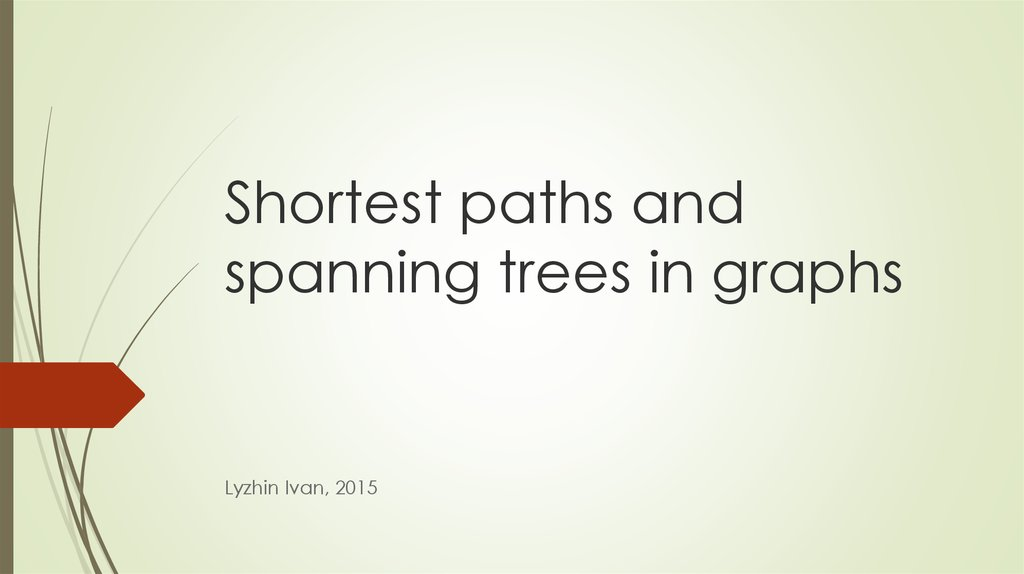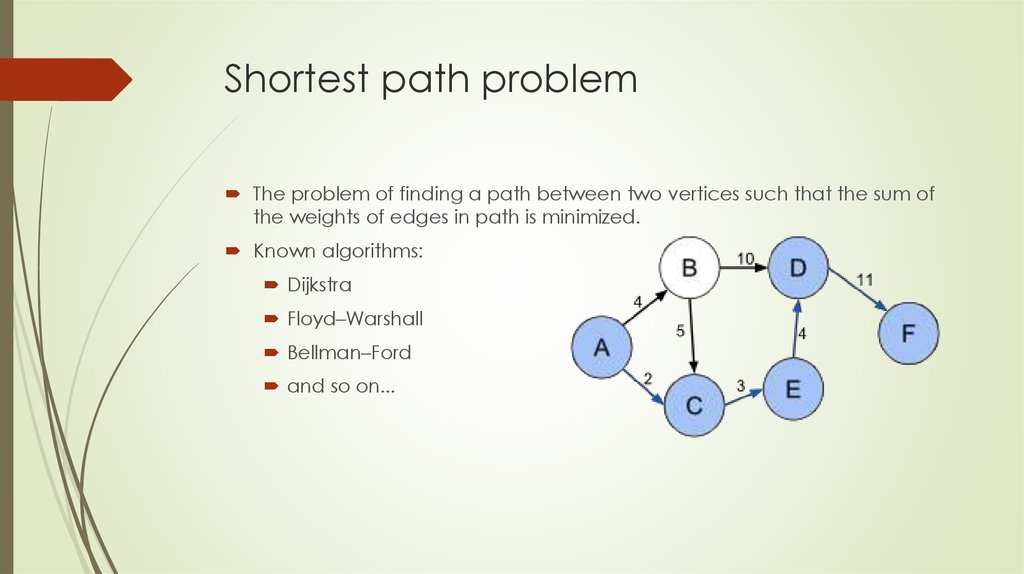# Shortest paths and spanning trees in graphs

## 2. Shortest paths and spanning trees in graphs

Lyzhin Ivan, 2015

## 3. Shortest path problem

The problem of finding a path between two vertices such that the sum of
the weights of edges in path is minimized.
Known algorithms:
Dijkstra
Floyd–Warshall
Bellman–Ford
and so on...

## 4. Dijkstra algorithm

1. There are two sets of vertices – visited and unvisited.
2. For visited vertices we know minimal distance from start. For unvisited
vertices we know some distance which can be not minimal.
3. Initially, all vertices are unvisited and distance to each vertex is INF. Only
distance to start node is equal 0.
4. On each step choose unvisited vertex with minimal distance. Now it’s
visited vertex. And try to relax distance of neighbors.
Complexity: trivial implementation O(|V|^2+|E|)
implementation with set O(|E|log|V|+|V|log|V|)

## 5. Trivial implementation

void dijkstra(int s)
{
vector<bool> mark(n, false);
vector<int> d(n, INF);
d[s] = 0;
for (int i = 0; i < n; ++i)
{
int u = -1;
for (int j = 0; j < n; ++j)
if (!mark[j] && (u == -1 || d[j] < d[u]))
u = j;
mark[u] = true;
for (v - сосед u)
d[v] = min(d[v], d[u] + weight(uv));
}
}

## 6. Implementation with set

void dijkstra(int s)
{
set<pair<int, int> > q; //(dist[u], u)
vector<int> dist(n, INF);
dist[s] = 0;
q.insert(mp(0, s));
while(!q.empty())
{
int cur = q.begin()->second;
q.erase(q.begin());
for(auto e : g[cur])
if(dist[e.first] > dist[cur]+e.second)
{
q.erase(mp(dist[e.first], e.first));
dist[e.first] = dist[cur] + e.second;
q.insert(mp(dist[e.first], e.first));
}
}
}

## 7. Implementation with priority queue

void dijkstra(int s)
{
priority_queue<pair<int, int> > q; //(dist[u], u)
vector<int> dist(n, INF);
dist[s] = 0;
q.push(mp(0, s));
while(!q.empty())
{
int cur = q.top().second;
int cur_d = -q.top().first; q.pop();
if(cur_d > dist[cur]) continue;
for(auto e : g[cur])
if(dist[e.first] > dist[cur]+e.second)
{
dist[e.first] = dist[cur] + e.second;
q.push(mp(-dist[e.first], e.first));
}
}
}

## 8. Floyd–Warshall algorithm

1. Initially, dist[u][u]=0 and for each edge (u, v): dist[u][v]=weight(u, v)
2. On iteration k we let use vertex k as intermediate vertex and for each pair of
vertices we try to relax distance.
dist[u][v] = min(dist[u][v], dist[u][k]+dist[k][v])
Complexity: O(|V|^3)

## 9. Implementation

void floyd_warshall()
{
vector<vector<int> > dist(n, vector<int>(n, INF));
for (int i = 0; i < n; ++i)
dist[i][i] = 0;
for (int i = 0; i < n; ++i)
for (auto e : g[i])
dist[i][e.first] = e.second;
for (int k = 0; k < n; ++k)
for (int i = 0; i < n; ++i)
for (int j = 0; j < n; ++j)
dist[i][j] = min(dist[i][j], dist[i][k] + dist[k][j]);
}

## 10. Bellman–Ford algorithm

|V|-1 iterations, on each we try relax distance with all edges.
If we can relax distance on |V| iteration then negative cycle exists in
graph
Why |V|-1 iterations? Because the longest way without cycles from one
node to another one contains no more |V|-1 edges.
Complexity O(|V||E|)

## 11. Implementation

void bellman_ford(int s)
{
vector<int> dist(n, INF);
dist[s] = 0;
for (int i = 0; i < n - 1; ++i)
for (auto e : edges)
dist[e.v] = min(dist[e.v], dist[e.u] + e.weight);
for (auto e : edges)
if (dist[e.v] > dist[e.u] + e.weight)
cout << "Negative cycle!" << endl;
}

## 12. Minimal spanning tree

A spanning tree T of an undirected graph G is a subgraph that includes all
of the vertices of G that is a tree.
A minimal spanning tree is a spanning tree and sum of weights is minimized.

## 13. Prim’s algorithm

1. Initialize a tree with a single vertex, chosen arbitrarily from the graph.
2. Grow the tree by one edge: of the edges that connect the tree to vertices
not yet in the tree, find the minimum-weight edge, transfer it to the tree
and try to relax distance for neighbors.
3. Repeat step 2 (until all vertices are in the tree).
Complexity: trivial implementation O(|V|^2+|E|)
implementation with set O(|E|log|V|+|E|)

## 14. Implementation

void prima()
{
set<pair<int, int> > q; //(dist[u], u)
vector<int> dist(n, INF);
dist = 0;
q.insert(mp(0, 0));
while (!q.empty())
{
int cur = q.begin()->second;
q.erase(q.begin());
for (auto e : g[cur])
if (dist[e.first] > e.second)
{
q.erase(mp(dist[e.first], e.first));
dist[e.first] = e.second;
q.insert(mp(dist[e.first], e.first));
}
}
}

## 15. Kruskal’s algorithm

Create a forest F (a set of trees), where each vertex in the graph is a
separate tree
Create a set S containing all the edges in the graph
While S is nonempty and F is not yet spanning:
remove an edge with minimum weight from S
if the removed edge connects two different trees then add it to the
forest F, combining two trees into a single tree
Complexity: trivial O(|V|^2+|E|log|E|)
with DSU O(|E|log|E|)

## 16. Trivial implementation

void trivial_kruskal()
{
vector<int> color(n);
for (int i = 0; i < n; ++i)
color[i] = i;
sort(edges.begin(), edges.end());
for(auto e : edges)
if(color[e.u]!=color[e.v])
{
int c1 = color[e.u];
int c2 = color[e.v];
for (int i = 0; i < n; ++i)
if (color[i] == c1)
color[i] = c2;
}
}

## 17. Implementation with DSU

void kruskal()
{
DSU dsu(n);
sort(edges.begin(), edges.end());
for(auto e : edges)
if(dsu.findSet(e.u)!=dsu.findSet(e.v))
{
dsu.unionSets(e.u, e.v);
}
}

## 18. Disjoint-set-union (DSU)

Two main operations:
Find(U) – return root of set, which contains U, complexity O(1)
Union(U, V) – join sets, which contain U and V, complexity O(1)
After creating DSU:
After some operations:

## 19. Implementation

struct DSU
{
vector<int> p;
DSU(int n) {
p.resize(n);
for (int i = 0; i < n; ++i)
p[i] = i;
}
int find(int u) {
return u == p[u] ? u : find(p[u]);
}
void merge(int u, int v) {
int pu = find(u);
int pv = find(v);
p[pv] = pu;
}
};

## 20. Path compression

When we go up, we can remember root of set for each vertex in path
int findSet(int u)
{
return u == p[u] ? u : p[u] = findSet(p[u]);
}

## 21. Union by size

DSU(int size)
{
p.resize(size);
sizes.resize(size, 1);
for (int i = 0; i < size; ++i)
p[i] = i;
}
int unionSets(int u, int v)
{
int pu = findSet(u);
int pv = findSet(v);
if (pu == pv) return;
if (sizes[pu] < sizes[pv])
swap(pu, pv);
p[pv] = pu;
sizes[pu] += sizes[pv];
}

https://en.wikipedia.org/wiki/Dijkstra%27s_algorithm
https://en.wikipedia.org/wiki/Floyd–Warshall_algorithm
https://en.wikipedia.org/wiki/Bellman–Ford_algorithm
https://en.wikipedia.org/wiki/Kruskal%27s_algorithm
https://en.wikipedia.org/wiki/Prim%27s_algorithm
https://en.wikipedia.org/wiki/Disjoint-set_data_structure
http://e-maxx.ru/algo/topological_sort